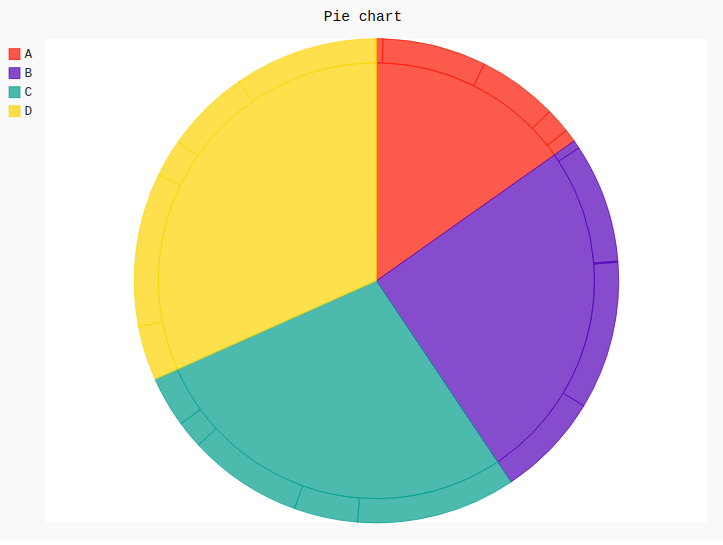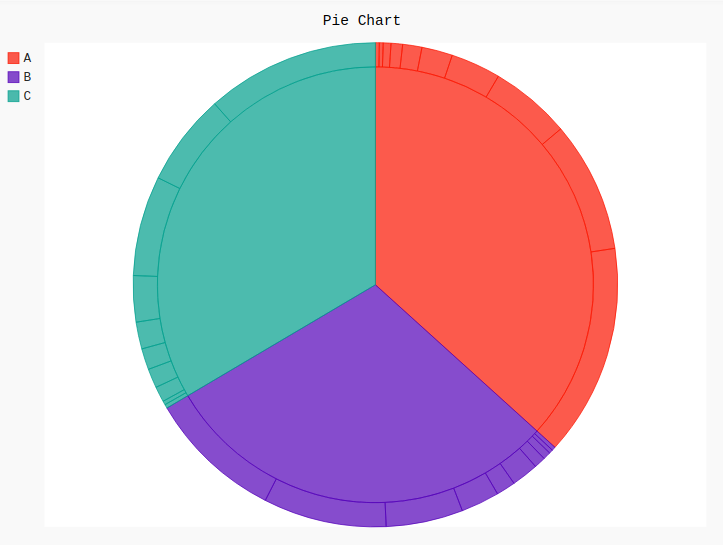GeeksforGeeks App
Open AppBrowser
Continue

# Multi-Series Pie Chart in Pygal

Pygal is a Python module that is mainly used to build SVG (Scalar Vector Graphics) graphs and charts. SVG is a vector-based graphics in the XML format that can be edited in any editor. Pygal can create graphs with minimal lines of code that can be easy to understand and write.

## Multi-Series Pie Chart

The multi-series pie chart is a chart that allows to present consistent and skewed structure in an affiliated pie like-structure. It presents the entire echelons in a single screen, which helps to present the pie more attractive and understanding. It can be created using the `Pie()` method of Pygal module.

Syntax:

`pie_chart = pygal.Pie()`

Example 1:

 `# importing pygal``import` `pygal``import` `numpy`` ` ` ` `# creating line chart object``pie_chart ``=` `pygal.Pie()`` ` `# naming the title``pie_chart.title ``=` `'Pie chart'`` ` ` ` `# random data``pie_chart.add(``'A'``, numpy.random.rand(``5``))``pie_chart.add(``'B'``, numpy.random.rand(``5``))``pie_chart.add(``'C'``, numpy.random.rand(``5``))``pie_chart.add(``'D'``, numpy.random.rand(``5``))`` ` `pie_chart`

Output:Example 2:

 `# importing pygal``import` `pygal`` ` ` ` `# creating pie_chart object``pie_chart ``=` `pygal.Pie()``pie_chart.title ``=` `'Pie Chart'`` ` `# random data``pie_chart.add(``"A"``, [``0``, ``1``, ``1``, ``2``, ``3``, ``5``, ``8``, ``13``, ``21``, ``34``, ``55``])``pie_chart.add(``"B"``, [``0``, ``1``, ``1``, ``2``, ``3``, ``7``, ``5``, ``10``, ``20``, ``32``, ``35``])``pie_chart.add(``"C"``, [``0``, ``1``, ``1``, ``4``, ``5``, ``5.5``, ``7``, ``12``, ``26``, ``24``, ``45``])`` ` `pie_chart`

Output:My Personal Notes arrow_drop_up